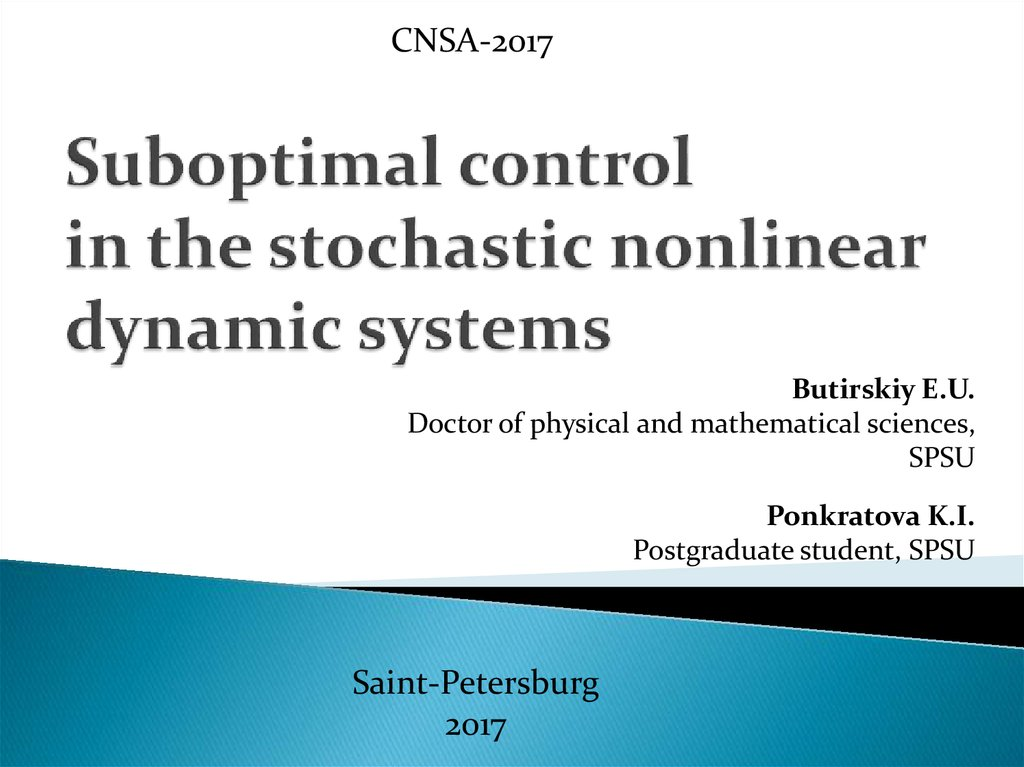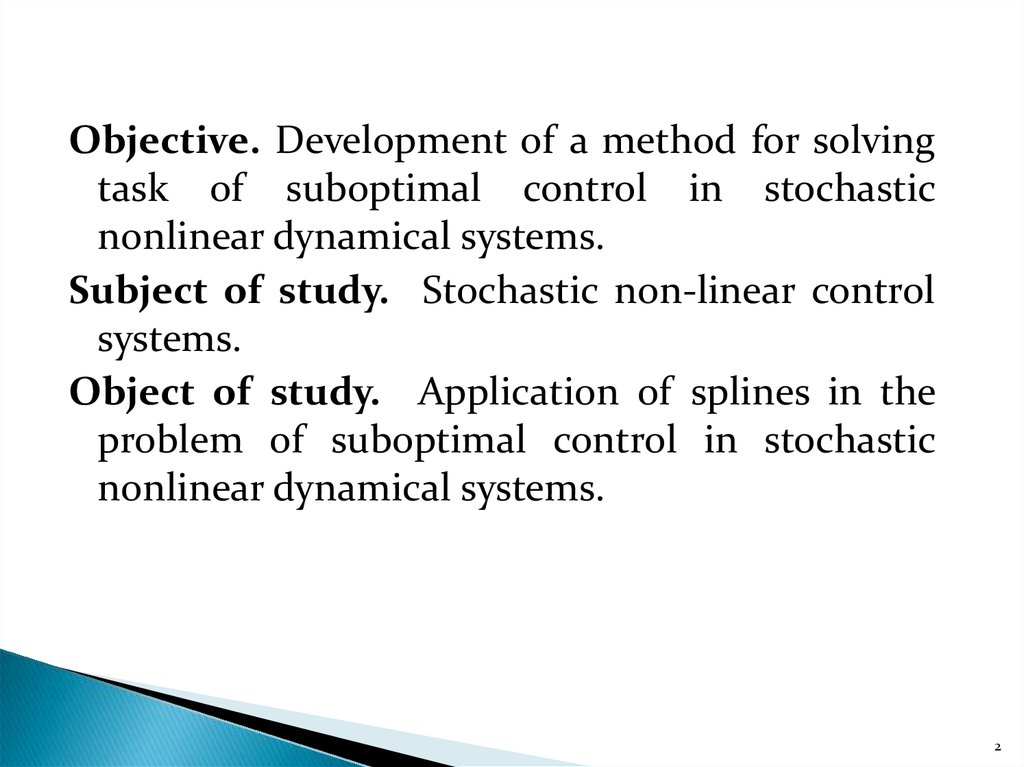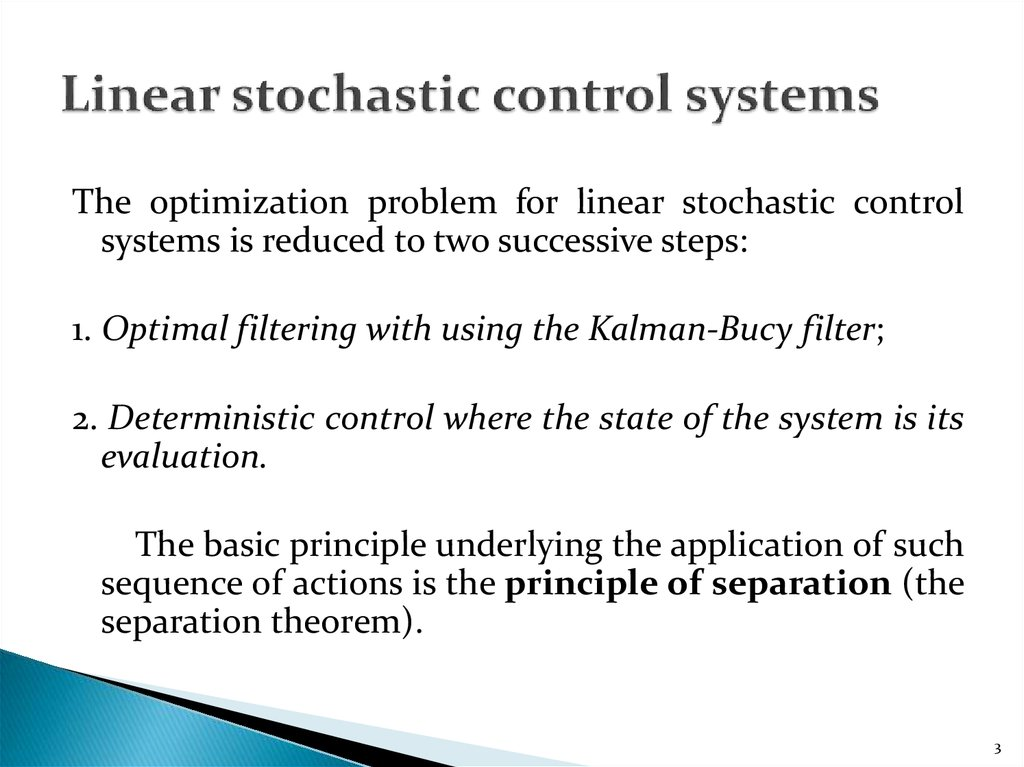# Suboptimal control in the stochastic nonlinear dynamic systems

## 1. Suboptimal control in the stochastic nonlinear dynamic systems

CNSA-2017
Butirskiy E.U.
Doctor of physical and mathematical sciences,
SPSU
Ponkratova K.I.
Saint-Petersburg
2017

## 2.

Objective. Development of a method for solving
task of suboptimal control in stochastic
nonlinear dynamical systems.
Subject of study. Stochastic non-linear control
systems.
Object of study. Application of splines in the
problem of suboptimal control in stochastic
nonlinear dynamical systems.
2

## 3. Linear stochastic control systems

The optimization problem for linear stochastic control
systems is reduced to two successive steps:
1. Optimal filtering with using the Kalman-Bucy filter;
2. Deterministic control where the state of the system is its
evaluation.
The basic principle underlying the application of such
sequence of actions is the principle of separation (the
separation theorem).
3

## 4. The principle of separation (the principle of stochastic equivalence)

In accordance with the principle of separation, the
problem of synthesizing a stochastic linear optimal
control system with incomplete information about
the state is divided into two:
The problem of synthesis of a linear optimal
observer;
Deterministic task of synthesis of an optimal
system.
4

## 5. The principle of separation (the principle of stochastic equivalence)

A stochastic linear optimal controller consists of a
linear optimal observer and a deterministic optimal
controller.
n1
n0
z
5

## 6. Nonlinear stochastic control systems

For nonlinear stochastic control systems, the separation
principle is not always possible, since it has not been
proved for them. On the other hand, the presence of
non-linearity leads to the fact that even if the formation
noise and observations are Gaussian, then the state is
non-Gaussian. The latter negatively affects the work of
the Kalman-Bucy filter it becomes not optimal and even
inoperative.
Therefore, approaches that allow us to find sub-optimal
estimates are relevant. In this connection, the use of
splines in the theory of control of stochastic systems is
promising and important both from the theoretical and
practical point of view.
6

## 7. Spline

is a function that is an algebraic polynomial on every
partial interval of interpolation, and on the whole given
interval is continuous along with several of its derivatives.
A spline curve is any composite curve formed by polynomial
sections that satisfy given conditions of continuity at the
boundaries of sections.
There are different types of splines used at the moment and
differing in the type of polynomials and certain specific
boundary conditions. The spline curve is given through a set
of coordinates of points, called control (reference), which
indicate the general shape of the curve. Then a piecewisecontinuous parametric polynomial function is selected from
these points (Fig. 1).
7

## 8. Linear spline

The linear spline is described by the following equation:
where the coefficients can be found by the formulas:
Linear spline has a number of distinctive advantages: it
is a significant reduction in computational costs and
universality.
8

## 9. Nonlinear stochastic control systems

Spline approximation of a nonlinear function f x
f x
S x
Figure 1. Representation of a function f x by linear
spline S x .
9

## 10. Nonlinear stochastic control systems

Let the control of the object and measurement models
be described by stochastic differential equations:
dx
f x V t u g1 t n1 t , x t0 x 0 ,
dt
z C t x g 2 t n 2 t , z t0 0,
(1)
where f (x) is a nonlinear smooth function (optional
condition).
We represent f(x) in the form of a spline of the first
order: f (x) S x A t x B t .
n
n
A t hij aij
, B t hij bij .
i 1
[ m m]
i 1
[ m 1]
10

## 11. Nonlinear stochastic control systems

With the approximation by splines of the expression (1)
takes the form:
dx
A t x B t V t u g1 t n1 t , x t0 x0 ,
dt
z C t x g 2 t n 2 t , z t0 0.
n
n
A t hij aij
, B t hij bij
,
i 1
[ m m]
i 1
[ m 1]
(2)
1 if x xi , xi 1 )
hi xi , xi 1
.
0
if
x
x
,
x
)
i i 1
11

## 12. Nonlinear stochastic control systems

We denote by
ztt0 = z , t0 t R1 t g1 t g1T t , R2 t g2 t gT2 t
Suppose that at the control at time t information about all
observations on the time interval [t0, t] is used.
The set of admissible controls form functions
u t u t , z tt0
t T , u t R ,
q
which depend on previous observations, for which the
system (1) has a unique solution.
12

## 13. Optimal control

Functional of quality of control
t
11 T
1
J x t S t x t uT t Q t u t dt xT t1 Λ x t1
2 t0
2
(3)
It is required to find a control u t , ztt from the set of
admissible ones that ensures the minimum of the
functional (3).
0
13

## 14. Sub-optimal control

t
u
t
,
z
Statement. Suboptimal control
t0
with the criterion of quality (3) has the form:
u t u t , z tt0 Q 1BT K 2 t x t .
dx
A t x B V t u K t z t C t x , x t0 m 0
dt
dK 2 t
dt
AT t K 2 t K 2 t A t K 2 t B t Q 1 t BT t K 2 t S t ,
K t Γ t CT t R 21 t K 2 t Λ.
dΓ t
dt
AΓ ΓAT ΓCT R 21CΓ R1
Γ t0 D0x
14

## 15. Sub-optimal control

K 2 t
- symmetric matrix of the gain factors of the
optimal controller,
K t - matrix of the gain factors of filter coefficients
dimension (n × m),
Γ t x t x t x t x t - covariance
matrix of estimation error,
T
x t x t z tt0 - estimation of the state vector of the
control object model from the results of observations.
15

## 16. Sub-optimal control

The system of equations on slide 14 is the Kalman-Bucy equation in
the representation of a nonlinear function in the form of linear
splines and describes the procedure for sub-optimal filtering and
control for a nonlinear stochastic system described by equations
(1). In general, the use of linear splines not only allowed us to solve
the problem, but also circumvented the restriction of the
separation theorem, which in principle was proved only for linear
systems (for nonlinear systems, the question remains open).
Splines allowed for each of the intervals to apply linear filtration
and the principle of separation.
As the results of the simulation show, the estimation of the state of
the system with the spline approximation very closely coincides
with the true value. Moreover, the number of the interval does not
influence the quality of the estimation.
Improve the quality by increasing the number of intervals. The
increase in the variance of observation noise is also adversely
affected in the case of optimal filtering and control, and in the case
of spline approximation.
16

## 17. Results of simulation of the filtration task

The state of the system x i and its estimate x i obtained
with the linear spline:
xi
xi
17

## 18. Conclusions

1. The use of splines allows one to solve problems in nonlinear
stochastic control systems (NSCS) that are defined not only by
a scalar but also by a matrix-vector equation.
2. The spline approximation has the global meaning, not local, as
in the case of Taylor series expansion.
3. Extrapolation of the obtained results to the case of parabolic
splines does not present difficulties, and the advantages of the
proposed approach in comparison with the known sub-optimal
methods of nonlinear filtration become even more significant.
4. The application of splines in HSCS of order higher than 2 does
not give special advantages in accuracy, but considerably
complicates the Kalman-Bucy filter and does not even allow it
to be realized.
18

## 19. Bibliography

 Burova I. G., Demyanovich Yu. K. Theory of
minimal splines. St. Petersburg State University,
(2001);
 Ogarkov M. A. Methods of statistical estimation of
parameters of random processes, - M .:
Energoatomizdat, (1990);
 Afanasiev V. N., Kolmanovsky V. B., Nosov V. R.
Mathematical theory of designing control systems. Moscow: Higher School, (1989).
19